# Pytorch入门教程10-数据预处理2020年10月9日07:15:58

2 6134字阅读20分26秒

## 简介

• 特征数据需要进行标准化, 将不同的特征都转换为0-1之间的数.
• 图像数据也需要从0-255转换为0-1之间, 同时图像数据还需要对大小进行剪裁等操作. 同时也可以通过图像变换来增加数据量, 提高模型的准确率和鲁棒性.

## torchvision.transforms.Compose

1. class ToTensor:
2.     # 将Numpy转换为tensor格式
3.     def __call__(self, X):
4.         return torch.from_numpy(X)
6.     def __call__(self, X):
7.         X = torch.tensor() + 2*X
8.         return X

1. # 定义预处理集合器

1. # 进行数据的测试
2. data = np.array([[1, 2, 3], [3,4,5]])
3. composed(data)
4. """
5. tensor([[ 3,  5,  7],
6.         [ 7,  9, 11]])
7. """

## 特征数据预处理

• 除了上面的方法, 我们可以使用sklearn中的数据预处理的方式对数据进行处理
• 我们也可以将其与dataset进行结合, 这样可以把数据导入和数据预处理结合在一起.

### 特征数据标准化

1. from sklearn.preprocessing import StandardScaler
2. sc = StandardScaler()
3. # 对特征进行标准化，标签不要标准化，因为标签只有 0 和 1
4. X_train = sc.fit_transform(X_train)
5. X_test = sc.transform(X_test)
6. X_train

### 与dataset结合使用

1. class TestDataset(Dataset):
2.     # 需要继承dataset数据集
3.     def __init__(self, transform):
4.         # I初始化数据
5.         xy = np.random.random((100, 13))*100
6.         self.n_samples = xy.shape # 样本的个数
7.         # 这里我们就不做Tensor的转换了，将其全部放入 transform 中
8.         self.x_data = xy[:, 1:]
9.         self.y_data = xy[:, 0].reshape(-1,1)
10.         # 数据预处理集合
11.         self.transform = transform
12.     # 返回 dataset[index]
13.     def __getitem__(selfindex):
14.         sample = self.x_data[index], self.y_data[index]
15.         if self.transform:
16.             # 数据预处理在这里(只对x进行预处理)
17.             sample = self.transform(sample)
18.         return sample
19.     def __len__(self):
20.         # 返回数据长度
21.         return self.n_samples

1. # 定义归一化操作
2. class Normalization:
3.     """这里是只对features进行归一化处理
4.     """
5.     def __call__(self, sample):
6.         inputs, targets = sample
7.         amin, amax = inputs.min(), inputs.max()  # 求最大最小值
8.         inputs = (inputs-amin)/(amax-amin)  # (矩阵元素-最小值)/(最大值-最小值)
9.         return inputs, targets
10. # 定义numpy转tensor
11. class ToTensor:
12.     def __call__(self, sample):
13.         inputs, targets = sample
14.         return torch.from_numpy(inputs), torch.from_numpy(targets)

1. # 定义 composed
2. composed = torchvision.transforms.Compose([Normalization(), ToTensor()])
3. # 初始化dataset的时候传入transform即可
4. dataset = TestDataset(transform=composed)

1. features_0, labels_0 = dataset
2. # 查看数据类型
3. print(type(features_0), type(labels_0))
4. """
5. <class 'torch.Tensor'> <class 'torch.Tensor'>
6. """
7. # 查看是否进行归一化
8. print(features_0) # 这里是进行归一化的结果
9. print(labels_0) # 这里是没有进行归一化的结果
10. """
11. tensor([0.0935, 0.2159, 0.9332, 0.0000, 0.0356, 0.3848, 0.4804, 0.0839, 0.1280,
12.         0.7919, 0.6031, 1.0000], dtype=torch.float64)
13. tensor([44.6151], dtype=torch.float64)
14. """

## 图像数据预处理

1. import torchvision.transforms as transforms
2. from PIL import Image
3. import matplotlib.pyplot as plt
4. from matplotlib.pyplot import imshow
5. %matplotlib inline
6. img = Image.open("20200804_141727_tnzpt18.jpg")
7. imshow(img)### 剪裁图像大小

1. # torchvision.transforms.CenterCrop（size）：从中心开始，裁剪给定大小的 PIL 图像
2. transform = transforms.CenterCrop((300, 400))
3. new_img = transform(img)
4. imshow(new_img)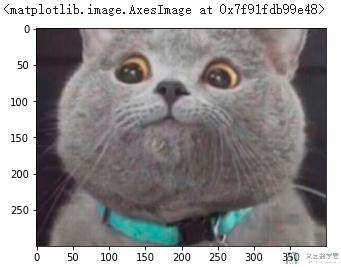### 图像的随机剪裁

1. #torchvision.transforms.RandomResizedCrop(size, scale,ratio,interpolation)
2. new_img = transforms.RandomResizedCrop((300, 400), scale=(0.08, 1.0), ratio=(0.75, 1.333333333), interpolation=2)(img)
3. imshow(new_img)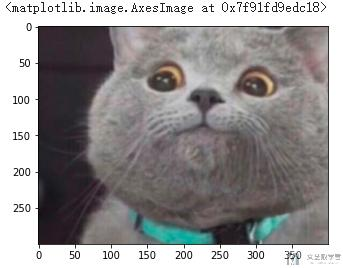### 改变图像亮度, 对比对和饱和度

1. # transforms.ColorJitter(brightness=0, contrast=0, saturation=0, hue=0)：
2. # 改变图片的亮度、对比度和饱和度
3. plt.subplot(221)
4. imshow(img) # 显示原始图片
5. # r随机改变亮度
6. my_img1 = transforms.ColorJitter((0.5, 0.6))(img)
7. plt.subplot(222)
8. imshow(my_img1)
9. # 随机改变对比度
10. my_img2 = transforms.ColorJitter(0, (0.5, 0.6))(img)
11. plt.subplot(223)
12. imshow(my_img2)
13. # 随机改变饱和度
14. my_img3 = transforms.ColorJitter(0, 0, (0.5, 0.6))(img)
15. plt.subplot(224)
16. imshow(my_img3)
17. plt.show()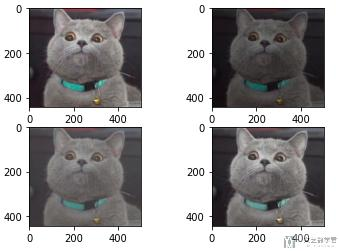### 转换为灰度图

• 如果返回的图像是单通道num_output_channels=1
• 如果返回的图像是3通道，其中num_output_channels=3, 且r == g == b

1. plt.subplot(131)
2. imshow(img) # 显示原始图片
3. my_img1 = transforms.Grayscale(1)(img)
4. plt.subplot(132)
5. imshow(my_img1, 'gray')
6. my_img2 = transforms.Grayscale(3)(img)
7. plt.subplot(133)
8. imshow(my_img2)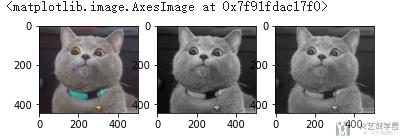### 图像的填充

2. # 使用给定的 pad 值将给定的 PIL 图像四处填充
3. plt.subplot(121)
4. imshow(img)
5. # 四周加黑色的边界
8. imshow(my_img)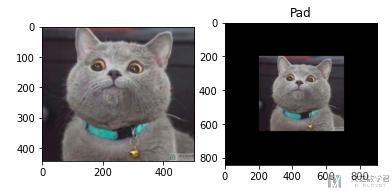### 中心仿射变换

1. # transforms.RandomAffine(degrees, translate=None, scale=None,
2. #                       shear=None, resample=False, fillcolor=0)：
3. # 保持图像中心不变的中心仿射变换(可以理解为不同程度的旋转，再在空余位置补 0)
4. my_img1 = transforms.RandomAffine(60)(img)
5. plt.subplot(221).set_title("rotate_only")
6. imshow(my_img1)
7. my_img2 = transforms.RandomAffine(60, translate=(0.3, 0.3))(img)
8. plt.subplot(222).set_title("rotate_translate")
9. imshow(my_img2)
10. my_img3 = transforms.RandomAffine(60, scale=(2.0, 2.1))(img)
11. plt.subplot(223).set_title("rotate_scale")
12. imshow(my_img3)
13. my_img4 = transforms.RandomAffine(0, shear=60)(img)
14. plt.subplot(224).set_title("shear_only")
15. imshow(my_img4)
16. plt.tight_layout()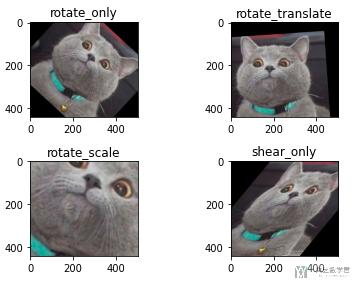### 翻转-RandomHorizontalFlip

1. plt.subplot(121)
2. img = Image.open("hymenoptera_data/train/ants/178538489_bec7649292.jpg")
3. imshow(img)
4. plt.subplot(122)
5. transform = torchvision.transforms.RandomHorizontalFlip()
6. new_img = transform(img)
7. print(new_img.size)
8. imshow(new_img)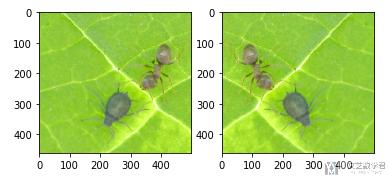• 微信公众号
• 关注微信公众号
•• QQ群
• 我们的QQ群号
•• 本文由 发表于 2020年10月9日07:15:58
• 转载请务必保留本文链接：https://mathpretty.com/12522.html